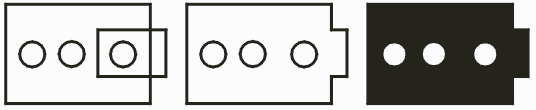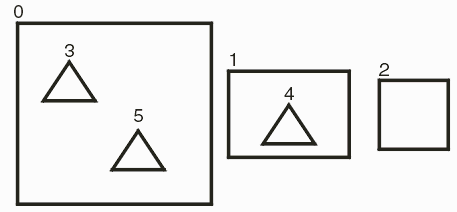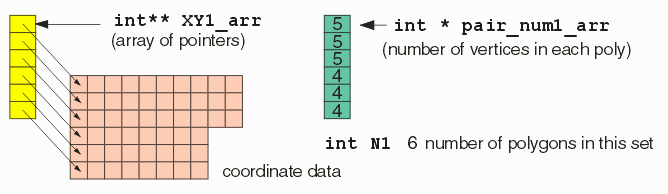### De-Embedding

De-embedding is the sorting of a collection of polygons into parents and children for the purposes of treating the parents as "dark" and the children as "clear." Many CAD systems use this approach to describe complicated polygonal areas with islands.

 Example 1 One parent with multiple childrenExample 2 Nested Parents and Children.### Handling Touching Polygons

Since we are sorting polygons as to whether they are parents or children the situation when two polygons have touching edges becomes a bit ambiguous. The rule is: If the edges of two polygons touch, or intersect then those two polygons will be unionized prior to any sorting process.

Example

In the illustration below, (a) two "child" polygons are touching; prior to sorting they are unionized(b) and the final results are shown (c).In the illustration below there is an intersection (a) with the "parent" polygon. The two rectangles are first booleanized (b) and then the dembedding sort is performed resulting in (c).Notice that this result is not entirely equivalent to the results one would obtain using a "scan line" function. The scan line would produce something more like the illustration below:### Function Syntax

```
int QisBool_DeEmbedding
( int** XY1_arr, int * pair_num1_arr, int N1,
int*** XY_arr, int** pair_num_arr, int * N,
void *bdll_handle );

```

Prior to calling the QisBool_DeEmbedding function the caller must first populate the input data set. The illustration below shows graphically how this is done. In this example, our input data set consists of 6 polygons:The number next to each polygon indicates the order that it appears in the array of pointers.

The Arrays

An array of pointers is passed - this array points into the memory where the coordinate data for each polygon has been placed. An array of integers indicates the number of vertices for each polygon. The integer N1 indicates how many polygons in the array.The De-Embedded Polygons

Due to the fact that some unionization may occur, the list of returned polygons is not necessarily identical to the list of input polygons -- therefore one must return the same sort of data structure -- i.e. an array of pointers to the XY coordinates, a list of vertex counts for each poly and an integer N, indicating how many polys are returned.

The primary difference is both in the order of returned polygons and how parent/children are marked.

First, the polygons are returned in the order parent,child,child,child ... parent,child,child where the children of a parent follow the parent. Of course, there may be no children for some parents.

Children are marked by their vertex count - a negative value for vertex indicates that this polygon is a child.